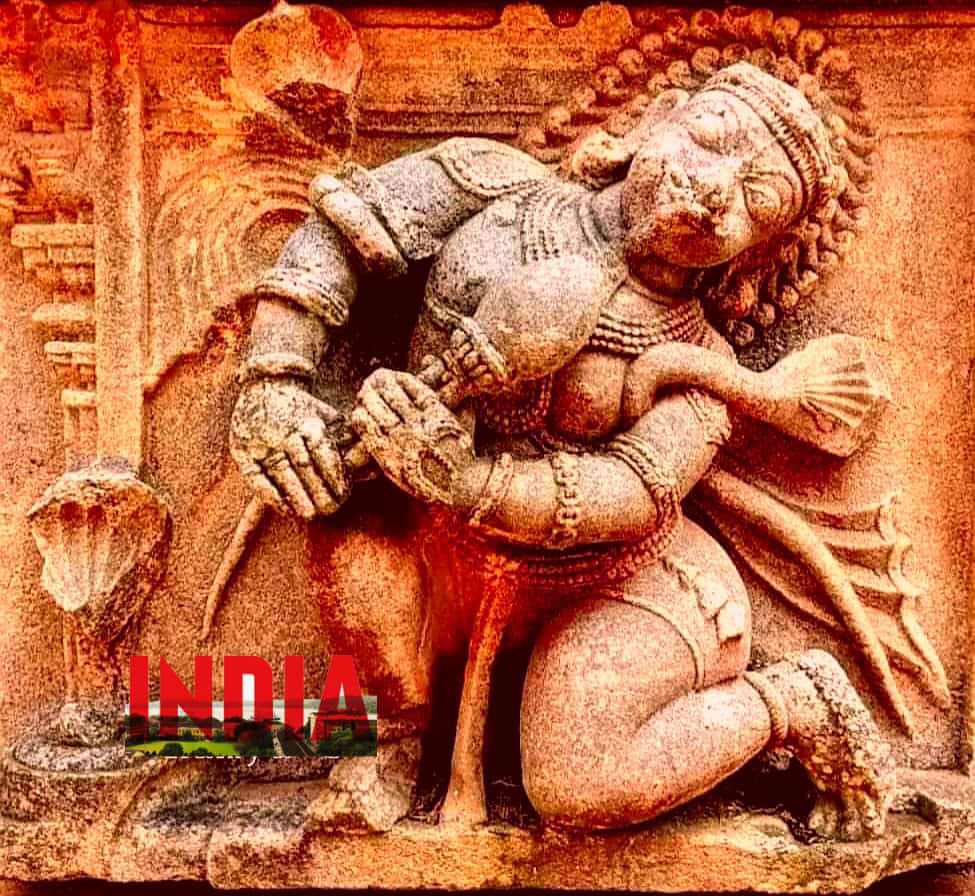## Tuesday, January 20, 2015

### Father of Binary System - Pingala the Genius MathematicianPingala is the author of Chandaḥśāstra (2nd century BCE)the earliest known Sanskrit treatise on prosody.He is identified either as the younger brother of Pāṇini or of Patañjali, the author of the Mahabhashya.His work, Chandaḥśāstra means science of meters, is a treatise on music and can be dated back to 2nd century BCE.

Pingala (in Chandaḥśāstra -8.23) has assigned the following combinations of zero and one to represent various numbers, much in the same way as the present day computer programming procedures.

0 0 0 0 numerical value = 1
1 0 0 0 numerical value = 2
0 1 0 0 numerical value = 3
1 1 0 0 numerical value = 4
0 0 1 0 numerical value = 5
1 0 1 0 numerical value = 6
0 1 1 0 numerical value = 7
1 1 1 0 numerical value = 8
0 0 0 1 numerical value = 9
1 0 0 1 numerical value = 10
0 1 0 1 numerical value = 11
1 1 0 1 numerical value = 12
0 0 1 1 numerical value = 13
1 0 1 1 numerical value = 14
0 1 1 1 numerical value = 15
1 1 1 1 numerical value = 16

Other numbers have also been assigned zero and one combinations likewise.

Pingala’s system of binary numbers starts with number one (and not zero). The numerical value is obtained by adding one to the sum of place values. In this system, the place value increases to the right, as against the modern notation in which it increases towards the left.

The procedure of Pingala system is as follows:

Divide the number by 2. If divisible write 1, otherwise write 0.
If first division yields 1 as remainder, add 1 and divide again by 2. If fully divisible, write 1, otherwise write 0 to the right of first 1.
If first division yields 0 as remainder that is, it is fully divisible, add 1 to the remaining number and divide by 2. If divisible, write 1, otherwise write 0 to the right of first 0.
This procedure is continued until 0 as final remainder is obtained.
Example to understand Pingala System of Binary Numbers :

Find Binary equivalent of 122 in Pingala System :

Divide 122 by 2. Divisible, so write 1 and remainder is 61. 1
Divide 61 by 2. Not Divisible and remainder is 30. So write 0 right to 1. 10
Add 1 to 61 and divide by 2 = 31.
Divide 31 by 2. Not Divisible and remainder is 16. So write 0 to the right. 100
Divide 16 by 2. Divisible and remainder is 8. So write 1 to right. 1001
Divide 8 by 2. Divisible and remainder is 4. So write 1 to right. 10011
Divide 4 by 2. Divisible and remainder is 2. So write 1 to right. 100111
Divide 2 by 2. Divisible. So place 1 to right. 1001111
Now we have 122 equivalent to 1001111.

Verify this by place value system : 1×1 + 0×2 + 0×4 + 1×8 + 1×16 + 1×32 + 1×64 = 64+32+16+8+1 = 121
By adding 1(which we added while dividing 61) to 121 = 122, which is our desired number.
In Pingala system, 122 can be written as 1001111.

Though this system is not exact equivalent of today’s binary system used, it is very much similar with its place value system having 20, 20, 21, 22, 22, 23, 24, 25, 26 etc used to multiple binary numbers sequence and obtain equivalent decimal number.

Reference : Chandaḥśāstra (8.24-25) describes above method of obtaining binary equivalent of any decimal number in detail.
These were used 1600 years before westeners/arabs copied binary system from India through trade and invasion.

We now use zero and one (0 and 1) in representing binary numbers, but it is not known if the concept of zero was known to Pingala— as a number without value and as a positional location.Pingala’s work also contains the Fibonacci number, called mātrāmeru, and now known as the Gopala–Hemachandra number. Pingala also knew the special case of the binomial theorem for the index 2, i.e. for (a + b) 2, as did his Greek contemporary Euclid.